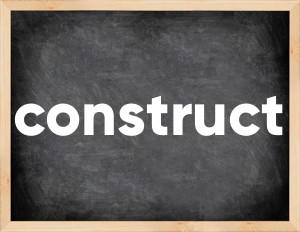# Construct past tenseThe English verb 'construct' is pronounced as [kənˈstrʌkt].
Related to: regular verbs.
3 forms of verb construct: Infinitive (construct), Past Simple - (constructed), Past Participle - (constructed).

## Here are the past tense forms of the verb construct

👉 Forms of verb construct in future and past simple and past participle.
❓ What is the past tense of construct.

## Construct: Past, Present, and Participle Forms

Base Form Past Simple Past Participle
construct [kənˈstrʌkt]

constructed [kənˈstrʌktɪd]

constructed [kənˈstrʌktɪd]

## What are the 2nd and 3rd forms of the verb construct?

🎓 What are the past simple, future simple, present perfect, past perfect, and future perfect forms of the base form (infinitive) 'construct'?

### Learn the three forms of the English verb 'construct'

• the first form (V1) is 'construct' used in present simple and future simple tenses.
• the second form (V2) is 'constructed' used in past simple tense.
• the third form (V3) is 'constructed' used in present perfect and past perfect tenses.

## What are the past tense and past participle of construct?

The past tense and past participle of construct are: construct in past simple is constructed, and past participle is constructed.

### What is the past tense of construct?

The past tense of the verb "construct" is "constructed", and the past participle is "constructed".

### Verb Tenses

Past simple — construct in past simple constructed (V2).
Future simple — construct in future simple is construct (will + V1).
Present Perfect — construct in present perfect tense is constructed (have/has + V3).
Past Perfect — construct in past perfect tense is constructed (had + V3).

### construct regular or irregular verb?

👉 Is 'construct' a regular or irregular verb? The verb 'construct' is regular verb.

## Examples of Verb construct in Sentences

•   It is possible to construct more realistic model. (Present Simple)
•   They constructed 26 houses. (Past Simple)
•   By now, nearly 650 houses have been constructed. (Present Perfect)
•   The author constructed the book from memories of her childhood. (Past Simple)
•   The firm contracted to construct the building. (Past Simple)
•   Construct a rectangle that has sides of equal length. (Present Simple)
•   They plan to construct a stable behind the house. (Present Simple)
•   Carol has constructed a new theory of marketing. (Present Perfect)
•   We want to construct a hut froom trees. (Present Simple)
•   The director constructs all the stories in the movie around one theme. (Present Simple)

Along with construct, words are popular interview and compare.

Verbs by letter: , , , , , , , , , , , , , , , , , , , , , , , , .